Question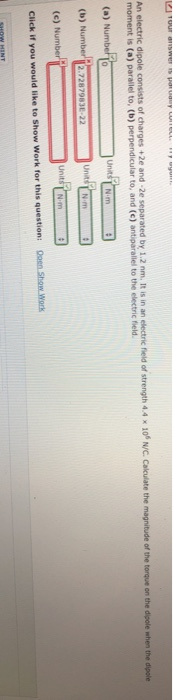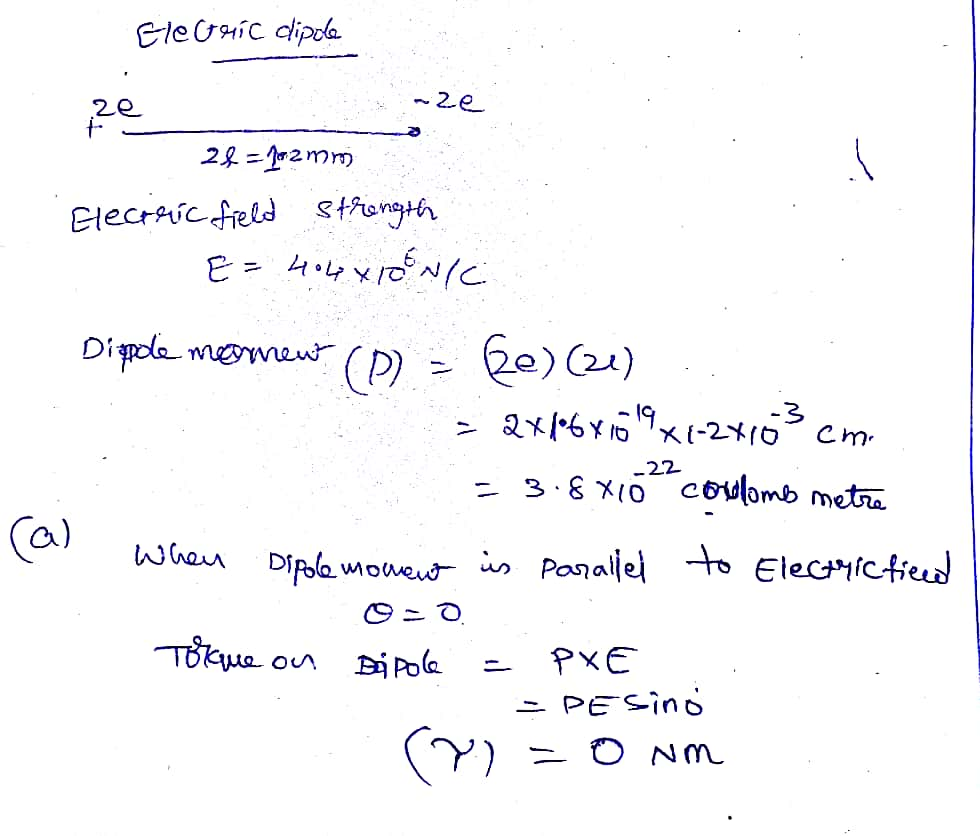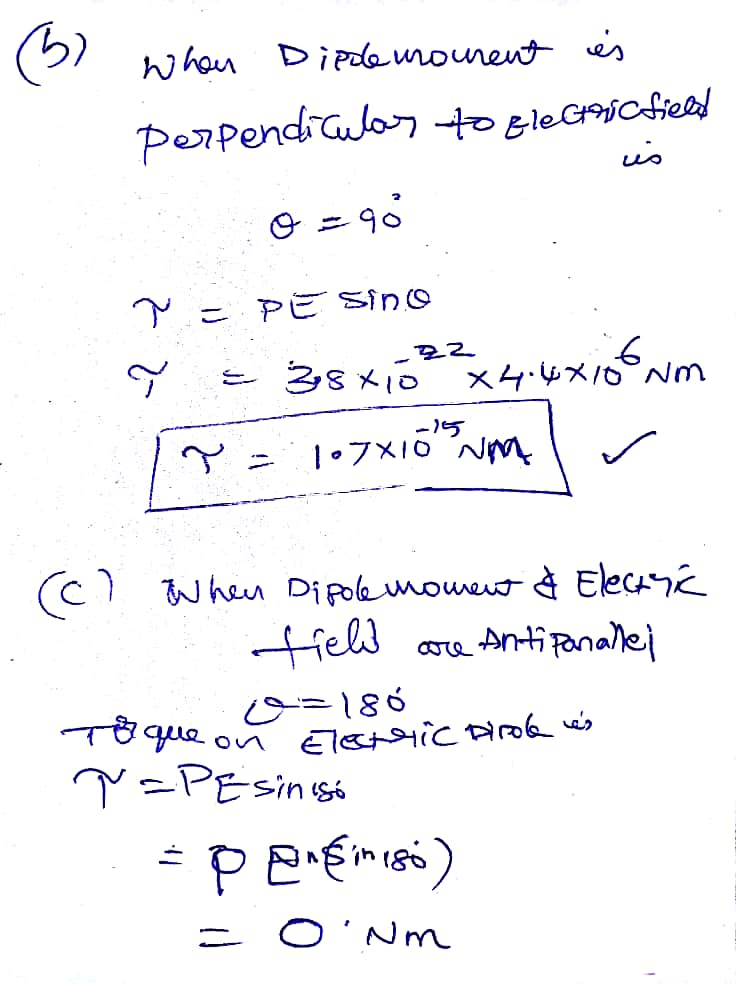#### Earn Coins

Coins can be redeemed for fabulous gifts.

Similar Homework Help Questions
• ### An electric dipole consists of charges +2e and -2e separated by 0.68 nm. It is in...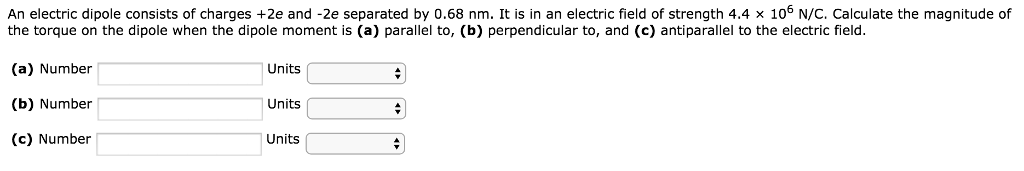An electric dipole consists of charges +2e and -2e separated by 0.68 nm. It is in an electric field of strength 4.4 × 106 N/C. Calculate the magnitude of the torque on the dipole when the dipole moment is (a) parallel to, (b) perpendicular to, and (c) antiparallel to the electric field. An electric dipole consists of charges +2e and-2e separated by 0.68 nm. It is in an electric field of strength 4.4 x 106 N/C. Calculate the magnitude of...

• ### An electric dipole consists of charges +2e and -2e separated by 0.90 nm. It is in...

An electric dipole consists of charges +2e and -2e separated by 0.90 nm. It is in an electric field of strength 3.2 × 106 N/C. Calculate the magnitude of the torque on the dipole when the dipole moment is (a) parallel to, (b) perpendicular to, and (c) antiparallel to the electric field.

• ### An electric dipole consists of charges +2e and -2e separated by 0.60 nm. It is in...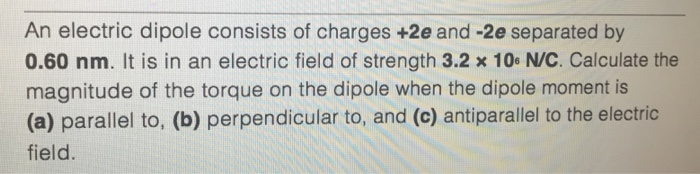An electric dipole consists of charges +2e and -2e separated by 0.60 nm. It is in an electric field of strength 3.2 times 10 N/C. Calculate the magnitude of the torque on the dipole when the dipole moment is (a) parallel to, (b) perpendicular to, and (c) antiparallel to the electric field.

• ### An electric dipole consists of charges +2e and-2e separated by 0.81 nm. It is in an...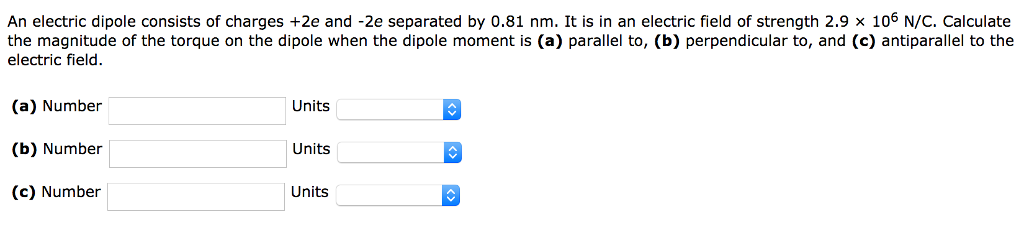An electric dipole consists of charges +2e and-2e separated by 0.81 nm. It is in an electric field of strength 2.9 × 106 N/C, Calculate the magnitude of the torque on the dipole when the dipole moment is (a) parallel to, (b) perpendicular to, and (c) antiparallel to the electric field. (a) Number (b) Number (c) Number Units Units Units

• ### Question7 An electric dipole consists of charges +2e and -2e separated by 0.98 nm. It is...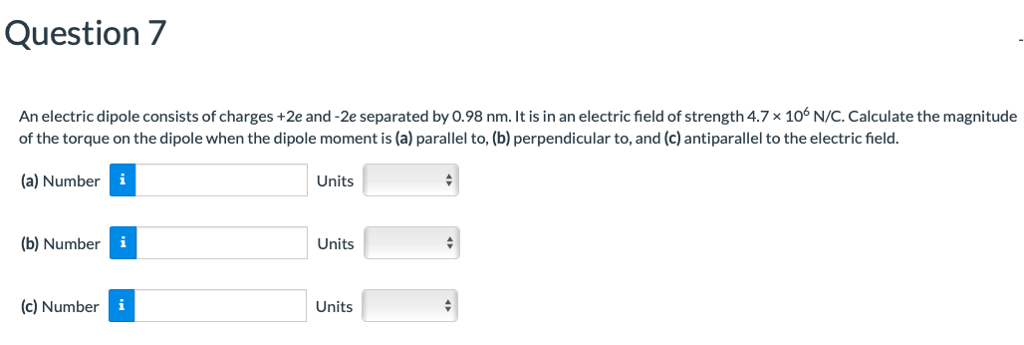Question7 An electric dipole consists of charges +2e and -2e separated by 0.98 nm. It is in an electric field of strength 4.7x 106 N/C. Calculate the magnitude of the torque on the dipole when the dipole moment is (a) parallel to, (b) perpendicular to, and (c) antiparallel to the electric field. (a) Number Units (b) Number Units (c) Number Units

• ### Chapter 22, Problem 056 An electric dipole consists of charges +2e and -2e separated by 0.91...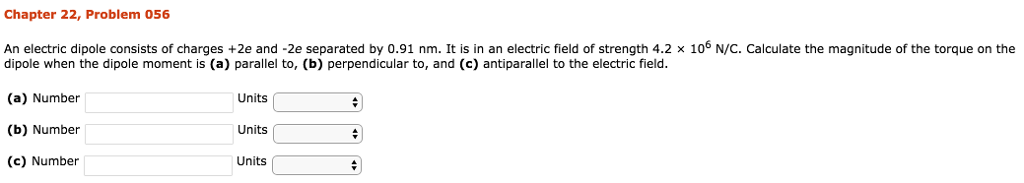Chapter 22, Problem 056 An electric dipole consists of charges +2e and -2e separated by 0.91 nm. It is in an electric field of strength 4.2 x 106 N/C. Calculate the magnitude of the torque on the dipole when the dipole moment is (a) parallel to, (b) perpendicular to, and (c) antiparallel to the electric field. (a) Number (b) Number (c) Number Units Units Units

• ### An electric dipole consists of charges +2e and -2e separated by 0.608 nm. It is in...

An electric dipole consists of charges +2e and -2e separated by 0.608 nm. It is in an electric field of strength 3.64×106 N/C. Calculate the magnitude of the torque on the dipole when the dipole moment is perpendicular to the electric field.

• ### Chapter 22, Problem 056 An electric dipole consists of charges +2e and-2e separated by 0.77 nm....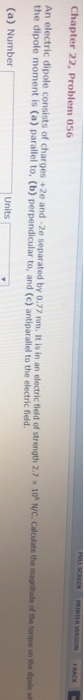Chapter 22, Problem 056 An electric dipole consists of charges +2e and-2e separated by 0.77 nm. It is in an electric field of strength 2.7 x 10s N/C the dipole moment is (a) parallel to, (b) perpendicular to, and (c) antiparallel to the electric field. (a) Number Units

• ### Magnitude of torque on an electric dipole

An electric dipole consists of charges +2e and -2e separated by 0.66 nm. It is in an electric field of strength 2.5 106 N/C. Calculate the magnitude of the torque onthe dipole when the dipole moment has the following orientations.(a) parallel to the field (N·m)(b) perpendicular to the field (N·m)(c) antiparallel to the field (N·m)

• ### An electric dipole consists of charges + 2e and , 2e separated by 0 82 nm....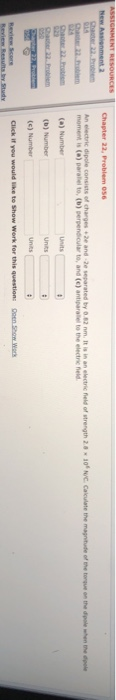An electric dipole consists of charges + 2e and , 2e separated by 0 82 nm. It as in an electric field of strength 2尋 moment is (a) paralel to, (b) perpendicular to, and (c) antiparallel to the electic ld 10.,uc calculate the magntade ofthe towe on the dpole when the dpole (a) Number (b) Number (c) Number Work for this question